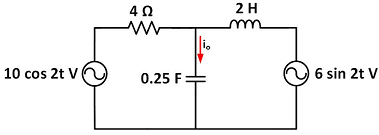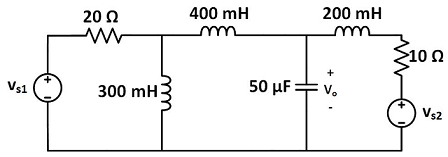### Find current in the circuit

Assignment Help Electrical Engineering
##### Reference no: EM132136853

Questions -

Problem 1 - Solve for i0 in Fig. using mesh analysis.Problem 2 - Use mesh analysis to find current i0 in the circuit.Problem 3 - Use mesh analysis to find v0 in the circuit. Let vs1 = 120 cos(100t+ 90o) V, vs2 = 80 cos(100t) V.#### Draw out the logic diagram of the multiplier

Design: draw out the logic diagram of the multiplier (e.g. see the logic diagram of a 2-bit and 2-bit multiplier illustrated in the Pre-lab and Background Information). Rememb

#### Consider the figure shown below. assume the length of lines

Consider the figure shown below. Assume the length of lines XY and YZ are equal and each relay covers 80% of length in its primary zone. If the fault occurs on the line XY at

#### Two milli-ammeters, with a full-scale current of 1 ma and 10

Two milli-ammeters, with a full-scale current of 1 mA and 10 mA, are connected in parallel and they read 0.5 mA and 2.5 mA respectively. Their internal resistances are in the

#### A 260/130v, 26kva single phase transformer has full load

A 260/130V, 26kVA single phase transformer has full load unity power factor efficiency of 94%. It is connected as an auto transformer to feed a load at 390V with input voltage

#### The current passing through a 10 ohm resistor in figure 1

The current passing through a 10 ohm resistor in figure-1 has the waveform shown in figure-2. The reading of the moving iron voltmeter connected across the resistor is

#### A 450 v 60 hz 8 pole 3-f induction motor runs at 873 rpm

A, 450 V, 60 Hz, 8 pole, 3-f induction motor runs at 873 rpm when driving a fan. The speed drops to 864 rpm when the motor reaches its final temperature. The increase in rotor

#### Find the area of the smaller zone determined by this section

A terrestrial globe is 20 in. in diameter. A plane is passed so as to cut from the globe a circular section 16 in. in diameter. Find the area of the smaller zone determined

#### During expansion 10 kj kg of heat is lost to the surrounding

In a turbine air expands from 5 bar, 520°C to 1 bar, 300°C. During expansion 10 kJ/kg of heat is lost to the surroundings which is at 0.98 bar, 20°C. Neglecting kinetic and po

### Write a Review